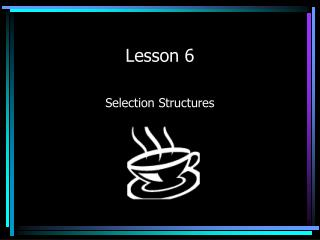DownloadDownload PresentationLesson 6

# Lesson 6

Télécharger la présentation## Lesson 6

- - - - - - - - - - - - - - - - - - - - - - - - - - - E N D - - - - - - - - - - - - - - - - - - - - - - - - - - -
##### Presentation Transcript

1. Lesson 6 Selection Structures

3. Simple Assignment Compound Addition Compound Subtraction Compound Multiplication Compound Division Compound Remainder = += -= *= /= %= Compound Assignment Operations (integers only)

4. x = x + 10 x = x – 10 x = x * 10 x = x / 10 x = x % 10 x += 10 x –= 10 x *= 10 x /= 10 x %= 10 Compound Assignment Equalities

5. Compound Assignments Translate the following statements to equivalent statements that use extended assignment operators: a. X = X *2; a. X *=2 b. Y %= 2 b. Y = Y % 2;

6. Math Class • The math class is quite extensive but we will concentrate a just a few of it’s properties:

7. Examples of Math Class Methods int m; double x; m = Math.abs(-7) // m equals 7 x = Math.abs(-7.5) // x equals 7.5 x = Math.pow(3.0,2.0) // x equals 3.0^2.0 = 9.0 x = Math.pow(16.0, .25) // x equals 16.0 ^ .25 = 2.0 m = Math.max(20,40) // m equals 40 m = Math.min(20,40) // m equals 20 m = (int) Math.round(4.27) // m equals 4

8. Round to two decimal places • (double) Math.round(answer*100)/100

9. Math Class //Given the area of a circle, compute its radius. double area = 10.0, radius; radius = Math.sqrt(area / Math.PI); Math.PI is accurate to about 17 decimal places

10. Random Class • The Random class has two methods that will generate a random integer or double.

11. Example of Random Class Methods Import java.util.Random; Random generator = new Random(); int i; double j; i = generator.nextInt(3); // would give a // random number 0,1, or 2. j = generator.nextDouble(); // would give a // random number // between 0 and 1

12. Control Structures • A control structure is simply a pattern for controlling the flow of a program module. • The three fundamental control structures of a structured programming language are sequence, selection, and iteration. • Sequence control structure is what you have been doing up until now. The second two is what we are going to take a look at next.

13. Selection/Iteration Structure • Selection and Iteration allow the flow of the program to be altered, depending on one or more conditions. • Selection is implemented by using a if, if/else, and switch statements. • Iteration is implemented by using the while, do/while, and for statements.

14. The IF statement • The if statement works much the same as a if/then statement in Visual Basic. • It uses relational operators to test if something is true or false. • If it is true, the program will execute the statement(s) within the if statement. • If it is false, the program will bypass the statements and continue with the statements following the if statement.

15. Syntax of the If Statement if (test expression) { statement1; statement2; statementn; } //this is an example of a compound statement

16. IF/ELSE Statement • The IF/ELSE statement works in the same manner as the if/then/else in Visual Basic. • It is considered a double-alternative statement. • If the expression evaluates as true, then the statements inside the if are executed. • If the expression evaluates as false, then the statements inside the else are executed.

17. Syntax for if/else if (test expression) { statement1; statement2; statementn; } else { statement1; statement2; statementn; } //example of compound statements

18. Relational Operators Relational operators allow two quantities to be compared. = = Equal to ! = Not equal to < Less than < = Less than or equal > Greater than > = Greater than or equal

19. Logical Operators

20. Logical Operators

21. Switch Statement • The switch statement works in the same manner as the case select statement in Visual Basic. • A selector variable is first evaluated to produce a value. • The selector is then compared to a series of cases. • If the selector value matches one of the case values, the corresponding case statements are executed.

22. Syntax for a Switch Statement switch (selector variable) //must be int or char { case case1value : case1statements; break; case case2value : case2statements; break; case casenvalue : case_N_statements; break; default : case exception statements; }

23. \b \t \n \” \’ \\ Backspace Tab Newline or line feed Double Quote Single Quote Backslash Escape Sequences

24. Bottoms Up On The Fourth Cup